Next: Coulomb's Law Up: Electrostatic Fields Previous: Laplace's Equation

Poisson's Equation

Poisson's equation is written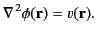(137)

Here, the function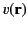is conventionally referred to as a source. Suppose that we have to solve Equation (137) over all space, subject to the boundary conditionas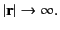(138)

We can achieve this task by searching for a so-called Green's function,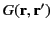, that satisfies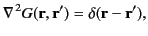(139)

subject to the boundary condition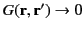as(140)

[Note that the source in Equation (137) is minus the source in the previously defined version of Poisson's equation, (36). Likewise, the Green's function (139) is minus the previously defined Green's function (41). These differences in sign are purely for the sake of convenience.] Once we have found the Green's function, the general solution to Equation (137), subject to the boundary condition (138), is given by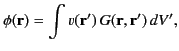(141)

where the integral is over all space. We can prove that this expression is indeed a solution to Equation (137) as follows: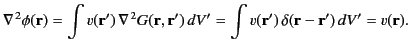(142)

Here, use has been made of Equations (23) and (24), as well as the fact thatand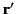are independent variables. From Equation (140), the expression forgiven in Equation (141) satisfies the boundary condition (138) provided that the volume integral on the right-hand side [of (141)] converges to a finite value.

According to Equation (25), a solution to Equation (139), subject to the boundary condition (140), is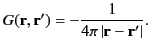(143)

Actually, we can prove that this is the only solution. Let there be two supposedly different functions,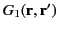and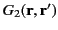, that both satisfy Equation (139), subject to the boundary condition (140). Let us form the difference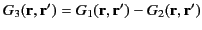. It follows that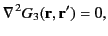(144)

subject to the boundary condition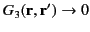as(145)

However, as we saw in Section 2.2, the only solution to the previous two equations is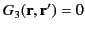(146)

for all(and). Hence, the functionsandare identical, and the Green's function (143) is unique. It follows from Equation (141) that the general solution to Poisson's equation, (137), subject to the boundary condition (138), is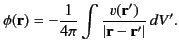(147)

Furthermore, this solution is unique.Next: Coulomb's Law Up: Electrostatic Fields Previous: Laplace's Equation
Richard Fitzpatrick 2014-06-27